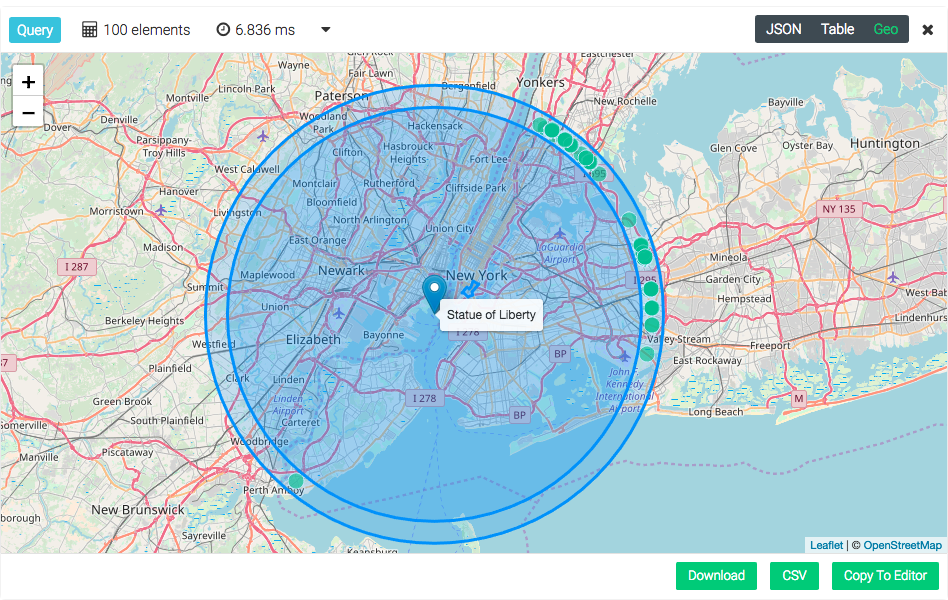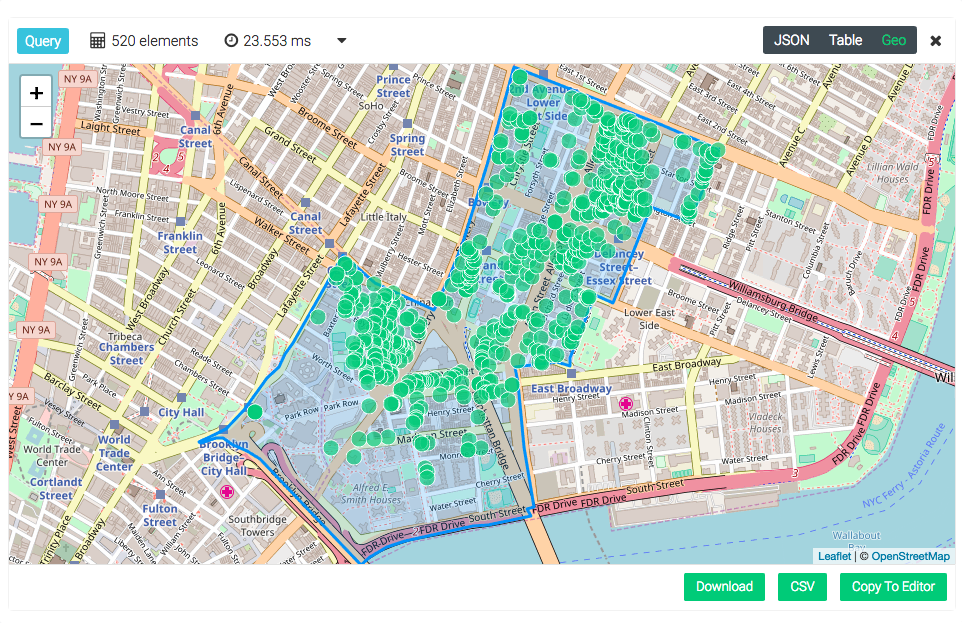# GeoJSON

GeoJSON is an open standard format for geographical features or data structures including properties and their spatial extends based on JSON. Back in the days it started as a community project by developers, but today it is fully specified and released by the IETF (RFC7946). GeoJSON uses a geographic coordinate reference system (WGS84) and units of decimal degrees for representing positions on earth with their longitude and latitude values.

GDN currently supports `Point`, `MultiPoint`, `LineString`, `MultiLineString`, `Polygon` and `MultiPolygon` as GeoJSON types.

A GeoJSON representation of a Point:

``{ "coordinates": [10.0, 20.0], "type": "Point" }``

Where 10.0 stands for the longitude value and 20.0 for the latitude value.

## GeoJSON Supported Index​

To create a geospatial index, which supports GeoJSON, on a collection named `restaurants`, use this command:

``restaurants.ensureIndex({  type: "geo",  fields: [ "location" ],  geoJson:true});``

The newly created index is expecting a valid GeoJSON object in each document inside the `location` property. A valid document would look like this:

``{  "location": {    "coordinates": [-73.97632519999999, 40.6748163 ],    "type": "Point"  },  "name": "Crab Spot Restaurant"}``

Invalid documents will be ignored. You are also able to create indices via the Web UI.

## GeoJSON Constructors​

Macrometa GDN provides following GeoJSON constructors as part of C8QL to help create GeoJSON types easily:

• GEO_LINESTRING()
• GEO_MULTILINESTRING()
• GEO_MULTIPOINT()
• GEO_POINT()
• GEO_POLYGON()
• GEO_MULTIPOLYGON()

### GEO_LINESTRING()​

To construct a `GeoJSON LineString`, you need atleast two longitude/latitude pairs.

Format:

`GEO_LINESTRING(points) → geoJson`

• points (array): number array of longitude/latitude pairs
• returns geoJson (object): a valid GeoJSON LineString

Example:

``  RETURN GEO_LINESTRING([    [35, 10], [45, 45]  ])``

Result:

``  [    {      "type": "LineString",      "coordinates": [        [          35,          10        ],        [          45,          45        ]      ]    }  ]``

### GEO_MULTILINESTRING()​

To construct a `GeoJSON MultiLineString`, you need atleast two elements consisting of valid line strings

Format:

`GEO_MULTILINESTRING(points) → geoJson`

• points (array): array of LineStrings
• returns geoJson (object): a valid GeoJSON MultiLineString

Example:

``  RETURN GEO_MULTILINESTRING([    [[100.0, 0.0], [101.0, 1.0]],    [[102.0, 2.0], [101.0, 2.3]]  ])``

Result:

``  [    {      "type": "MultiLineString",      "coordinates": [        [          [            100,            0          ],          [            101,            1          ]        ],        [          [            102,            2          ],          [            101,            2.3          ]        ]      ]    }  ]``

### GEO_MULTIPOINT()​

To construct a `GeoJSON LineString`, you need atleast two longitude/latitude pairs.

Format:

`GEO_MULTIPOINT(points) → geoJson`

• points (array): number array of longitude/latitude pairs
• returns geoJson (object): a valid GeoJSON Point

Example:

``  RETURN GEO_MULTIPOINT([    [35, 10], [45, 45]  ])``

Result:

``  [    {      "type": "MultiPoint",      "coordinates": [        [          35,          10        ],        [          45,          45        ]      ]    }  ]``

### GEO_POINT()​

To construct a `GeoJSON Point`, you need a longitude and latitude.

Format:

`GEO_POINT(longitude, latitude) → geoJson`

• longitude (number): the longitude portion of the point
• latitude (number): the latitude portion of the point
• returns geoJson (object): a GeoJSON Point

Example:

``  RETURN GEO_POINT(1.0, 2.0)``

Result:

``  [    {      "type": "Point",      "coordinates": [        1,        2      ]    }  ]``

### GEO_POLYGON()​

To construct a `GeoJSON Polygon`, you need at least one array representing a loop. Each loop consists of an array with at least three longitude/latitude pairs. The first loop must be the outermost, while any subsequent loops will be interpreted as holes.

• points (array): array of (arrays of) longitude/latitude pairs
• returns geoJson (object|null): a valid GeoJSON Polygon

Format:

`GEO_POINT(longitude, latitude) → geoJson`

• longitude (number): the longitude portion of the point
• latitude (number): the latitude portion of the point
• returns geoJson (object): a GeoJSON Point

Example: Simple Polygon

Query:

``  RETURN GEO_POLYGON([    [0.0, 0.0], [7.5, 2.5], [0.0, 5.0]  ])``

Result:

``  [    {      "type": "Polygon",      "coordinates": [        [          [            0,            0          ],          [            7.5,            2.5          ],          [            0,            5          ]        ]      ]    }  ]``

Example: Advanced Polygon with a hole inside

Query:

``  RETURN GEO_POLYGON([    [[35, 10], [45, 45], [15, 40], [10, 20], [35, 10]],    [[20, 30], [35, 35], [30, 20], [20, 30]]  ])``

Result:

``  [    {      "type": "Polygon",      "coordinates": [        [          [            35,            10          ],          [            45,            45          ],          [            15,            40          ],          [            10,            20          ],          [            35,            10          ]        ],        [          [            20,            30          ],          [            35,            35          ],          [            30,            20          ],          [            20,            30          ]        ]      ]    }  ]``

### GEO_MULTIPOLYGON()​

To construct a `GeoJSON MultiPolygon`, you need at least two Polygons inside. See GEO_POLYGON() for the rules of Polygon construction.

Format:

`GEO_MULTIPOLYGON(polygons) → geoJson`

• polygons (array): array of arrays of array of longitude/latitude pairs
• returns geoJson (object|null): a valid GeoJSON MultiPolygon

Example: MultiPolygon comprised of a simple Polygon and a Polygon with hole

``  RETURN GEO_MULTIPOLYGON([    [        [[40, 40], [20, 45], [45, 30], [40, 40]]    ],    [        [[20, 35], [10, 30], [10, 10], [30, 5], [45, 20], [20, 35]],        [[30, 20], [20, 15], [20, 25], [30, 20]]    ]  ])``

Result:

``  [    {      "type": "MultiPolygon",      "coordinates": [        [          [            [              40,              40            ],            [              20,              45            ],            [              45,              30            ],            [              40,              40            ]          ]        ],        [          [            [              20,              35            ],            [              10,              30            ],            [              10,              10            ],            [              30,              5            ],            [              45,              20            ],            [              20,              35            ]          ],          [            [              30,              20            ],            [              20,              15            ],            [              20,              25            ],            [              30,              20            ]          ]        ]      ]    }  ]``

## GeoJSON Functions​

The following helper functions can use geo indexes, but do not have to in all cases. You can use all of these functions in combination with each other, and if you have configured a `geo index` it may be utilized

This sections introduces each function with a small example snippet:

### DISTANCE()​

Calculate the distance between two arbitrary coordinates in meters (as birds would fly). The value is computed using the haversine formula, which is based on a spherical Earth model. It’s fast to compute and is accurate to around 0.3%, which is sufficient for most use cases such as location-aware services.

Format:

`DISTANCE(latitude1, longitude1, latitude2, longitude2) → distance`

• latitude1 (number): the latitude portion of the first coordinate
• longitude1 (number): the longitude portion of the first coordinate
• latitude2 (number): the latitude portion of the second coordinate
• longitude2 (number): the longitude portion of the second coordinate
• returns distance (number): the distance between both coordinates in meters

Examples:

``  // Distance from Brandenburg Gate (Berlin) to headquarters (Cologne)  DISTANCE(52.5163, 13.3777, 50.9322, 6.94) // 476918.89688380965 (~477km)``
``  // Sort a small number of documents based on distance to Central Park (New York)  FOR doc IN doc // e.g. documents returned by a traversal    SORT DISTANCE(doc.latitude, doc.longitude, 40.78, -73.97)    RETURN doc``

### GEO_DISTANCE()​

Return the distance between two GeoJSON objects, measured from the centroid of each shape. For a list of supported types see the geo index page.

Format:

`GEO_DISTANCE(geoJsonA, geoJsonB, ellipsoid) → distance`

• geoJsonA (object): first GeoJSON object
• geoJsonB (object): second GeoJSON object
• ellipsoid (string, optional): reference ellipsoid to use. Supported are "sphere" (default) and "wgs84".
• returns distance (number): the distance between the centroid points of the two objects on the reference ellipsoid

Examples:

``  LET polygon = {    type: "Polygon",    coordinates: [[[-11.5, 23.5], [-10.5, 26.1], [-11.2, 27.1], [-11.5, 23.5]]]  }  FOR doc IN collectionName    LET distance = GEO_DISTANCE(doc.geometry, polygon) // calculates the distance    RETURN distance``

Return all `restaurants` with a maximum distance of 30km starting from the Statue of Liberty.

``LET statueOfLiberty = GEO_POINT(-74.044500, 40.689306)FOR restaurant IN restaurants  FILTER GEO_DISTANCE(statueOfLiberty, restaurant.location) <= 30000  RETURN restaurant.location``

Return all restaurants with a distance between 25km and 30km starting from the Statue of Liberty.

``LET statueOfLiberty = GEO_POINT(-74.044500, 40.689306)FOR restaurant IN restaurants  FILTER GEO_DISTANCE(statueOfLiberty, restaurant.location) <= 30000  FILTER GEO_DISTANCE(statueOfLiberty, restaurant.location) >= 25000  RETURN restaurant.location``Return 100 nearest restaurants sorted by their distance starting from the Statue of Liberty.

``FOR restaurant IN restaurants  LET statueOfLiberty = GEO_POINT(-74.044500, 40.689306)  SORT GEO_DISTANCE(statueOfLiberty, restaurant.location) ASC  LIMIT 100  RETURN restaurant.location``

### GEO_CONTAINS()​

Checks whether the GeoJSON object geoJsonA fully contains geoJsonB (Every point in B is also in A). The object geoJsonA has to be of type Polygon or MultiPolygon, other types are not supported because containment is ill defined. This function can be optimized by a S2 based geospatial index.

Format:

`GEO_CONTAINS(geoJsonA, geoJsonB) → bool`

• geoJsonA (object): first GeoJSON object or coordinate array (in longitude, latitude order)
• geoJsonB (object): second GeoJSON object or coordinate array (in longitude, latitude order)
• returns bool (bool): true when every point in B is also contained in A, false otherwise

Example:

First get the document representing the district “Chinatown”. Then iterate and filter trough all available restaurants and only show those, which are located inside “Chinatown”.

``FOR n IN neighborhoods  FILTER n.name == "Chinatown"  LET chinatown = n  FOR restaurant IN restaurants    FILTER GEO_CONTAINS(chinatown.geometry, restaurant.location)    RETURN restaurant.location``### GEO_AREA()​

Return the area for a polygon or multi-polygon on a sphere with the average Earth radius, or an ellipsoid. For a list of supported types see the geo index page.

Format:

`GEO_AREA(geoJson, ellipsoid) → area`

• geoJson (object): a GeoJSON object
• ellipsoid (string, optional): reference ellipsoid to use. Supported are "sphere" (default) and "wgs84".
• returns area (number): the area in square meters of the polygon

Example:

``  LET polygon = {    type: "Polygon",    coordinates: [[[-11.5, 23.5], [-10.5, 26.1], [-11.2, 27.1], [-11.5, 23.5]]]  }  RETURN GEO_AREA(polygon, "wgs84")``

### GEO_INTERSECTS()​

Checks whether the GeoJSON object geoJsonA intersects with geoJsonB (i.e. at least one point in B is also A or vice-versa). This function can be optimized by a S2 based geospatial index.

Format:

`GEO_INTERSECTS(geoJsonA, geoJsonB) → bool`

• geoJsonA (object): first GeoJSON object
• geoJsonB (object): second GeoJSON object.
• returns bool (bool): true if B intersects A, false otherwise

Example:

Define some area in NYC which potentially covers more neighborhoods. Then return all neighborhoods which will intersect with the defined area (Polygon).

``  LET someAreaInNYC = GEO_POLYGON([    [-74.02587890625, 40.70536767492135],    [-73.97335052490234, 40.71135347314246],    [-73.90434265136719, 40.797957124643666],    [-73.98193359375, 40.814328907637126],    [-74.02587890625, 40.70536767492135]  ])  FOR n IN neighborhoods    FILTER GEO_INTERSECTS(someAreaInNYC, n.geometry)    RETURN n.geometry``

### GEO_EQUALS()​

Checks whether two GeoJSON objects are equal or not. For a list of supported types see the geo index page.

Format:

`GEO_EQUALS(geoJsonA, geoJsonB) → bool`

• geoJsonA (object): first GeoJSON object
• geoJsonB (object): second GeoJSON object.
• returns bool (bool): true for equality.

Examples:

Below example checks whether the two geo-polygon objects are equal or not.

``  LET polygonA = GEO_POLYGON([    [-11.5, 23.5], [-10.5, 26.1], [-11.2, 27.1], [-11.5, 23.5]  ])  LET polygonB = GEO_POLYGON([    [-11.5, 23.5], [-10.5, 26.1], [-11.2, 27.1], [-11.5, 23.5]  ])  RETURN GEO_EQUALS(polygonA, polygonB) // true    LET polygonA = GEO_POLYGON([    [-11.1, 24.0], [-10.5, 26.1], [-11.2, 27.1], [-11.1, 24.0]  ])  LET polygonB = GEO_POLYGON([    [-11.5, 23.5], [-10.5, 26.1], [-11.2, 27.1], [-11.5, 23.5]  ])  RETURN GEO_EQUALS(polygonA, polygonB) // false``

Below example checks whether the two geo-point objects are equal or not.

``  LET A = GEO_POINT(1.0, 2.0)  LET B = GEO_POINT(3.0, 4.0)  RETURN {    "AA": GEO_EQUALS(A, A), // true    "AB": GEO_EQUALS(A, B) // false  }``